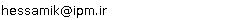••••••••## “Khodabakhsh Hessami Pilehrood”Tel:  (+98)(381)4424419
Fax:  (+98)(381)4424419
Email:### IPM Positions

Non Resident Researcher (non-resident), School of Mathematics
(2010 - 2012 )

### Past IPM Positions

Associate Researcher (non-resident), School of Mathematics
(2006 - 2009)
Associate Researcher (non-resident), School of Mathematics
(2004 - 2006)

Associate Researcher (non-resident), School of Mathematics
(2002 - 2003)

### Non IPM Affiliations

Associate Professor of Shahrekord University

### Research Interests

Number Theory in general, Diophantine Equations, Diophantine Approximations.

### Research Activities

Problem 1. It is well known that every rational number is a sum of three rational cubes. Thus it is an interesting question to determine the rational numbers which are sums of two rational cubes, or if we prefer, the integers A for which the equation
?

 x3+y3=Az3
(1)

is solvable in integers.

This equation has attracted the attention of many mathematicians over a period of years. Euler was first who proved that the equation (1) is impossible if a=1 and a=4, and that x=?y if a=2. Fundamental contribution to the investigation of such kind equations was made by Sylvester, van der Corput, Delone and Faddeev. Author proved that the equation
?

 x3+y3=pqz3

has no solutions in nonzero integers x, y, z if ?p, q are primes of special kind.

Note that a very interesting question for this problem is a search of other values of a for which the equation (1) has no solutions in nonzero integers x, y, z.

Problem 2. To investigate Diophantine equation
?

 xp-yq=k,

where the unknowns x,?y,?p and q are integers all ? 2, in some particular cases. In general case conjecture Pillai says that this equation has only finitely many solutions (x, y, p, q). This means that in the increasing sequence of perfect powers xp, with x ? 2 and p ? 2 the difference between two consecutive terms tends to infinity. It is not even known that for, say, k=2, Pillai''s equation has only finitely many solutions. A related open question is whether the number 6 occurs as a difference between two perfect powers: Is there a solution to the Diophantine equation xp-yq=6?

### Present Research Project at IPM

Diophantine equation

### Related Papers

 1. Bivariate identities for values of the Hurwitz zeta function and supercongruencesElectron. J. Combin. 18 (2012), #P35  [abstract]
 2. On a continued fraction expansion for Euler's constantJ. Number Theory  (Accepted) [abstract]
 3. Rational approximations for values of Bell polynomials at points involving Euler s constant and zeta valuesAustral. Math. Soc. (Accepted) [abstract]
 4. Rational approximations for values of the digamma function and a denominators conjectureMath. Notes (Accepted) [abstract]
 5. A q-analogue of the bailey-Borwein-Bradley identityJournal of Symbolic Computation 46 (2011), 699-711  [abstract]
 6. Vacca-type series for values of the generalized Euler constant function and its derivativeJournal of Integer Sequences 13 (2010), 10.7.3  [abstract]
 7. Rational approximations for the quotient of gamma valuesIndag. Math. (N.S.) 20 (2009), 583-601  [abstract]
 8. An Apery-like continued fraction for πcothπJ. Difference Equ. Appl. (Accepted) [abstract]
 9. Generating function identities for ζ(2n+2), zeta(2n+3) via the WZ methodElectron. J. Combin. 15 (2008), 1-9  [abstract]
 10. On a conjecture of ErdosMath. Notes 83 (2008), 281-284  [abstract]
 11. Infinite sums as linear combinations of polygamma functionsActa Arith. 130 (2007), 231-254  [abstract]
 12. Apéry-like recursion and continued fraction for π coth π ( In: Diophantische approximationen)[abstract]
 13. On conditional irrationality measures for values of the digamma fuctionJ. Number Theory 123 (2007), 241-253  [abstract]
 14. Arithmetical properties of some series with logarithmic coefficientMath. Z. 255 (2007), 117-131  [abstract]
 15. Irrationality of the sums of zeta valuesMath. Notes 79 (2006), 607-618  [abstract]
 16. On sums of two rational cubesIndian J. Pure Appl. Math. 36 (2006), 707-717  [abstract]
 17. Irrationality of certain numbers that contain values of the di- and trilogarithmMath. Z. 254 (2006), 299-313  [abstract]
 18. On the diophantine equation x2+3=pynIndian J. Pure Appl. Math. 36 (2005), 431-439  [abstract]
 19. Lower Bounds for linear forms in values of polylogarithmsMath. Notes 77 (2005), 573-579  [abstract]
 20. Criteria for irrationality of generalized Euler's constantJ. Number Theory 108 (2004), 169-185  [abstract]
 [Back]
scroll left or right